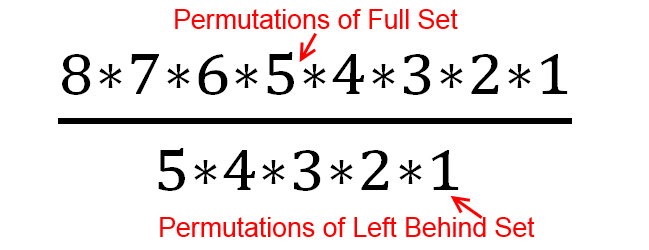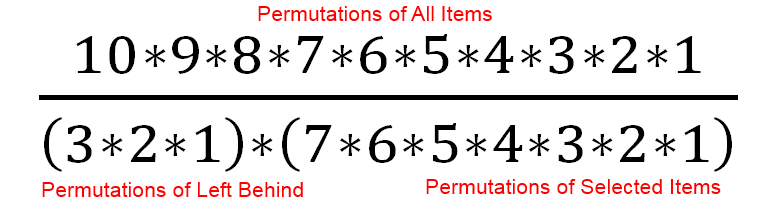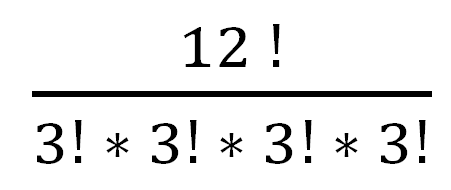# Permutations And Combinations Simplified

Permutations and combinations are an essential part of statistics. They show up in a ton of different places when you are finding the probability of anything. But it can be hard to remember the exact formula for a permutation or a combination when you need it without looking it up.   This post will show you an easy, intuitive way to understand permutations and combinations so that you only need to remember one thing, and the rest you can just calculate when you need it.

## Permutations and Combinations Basics

Permutations and combinations are a way of determining how many different possibilities of something there are.

Permutations are what you use when the order matters. For instance, if 8 people are racing in a track meet, and you want to find the different ways they could get 1st, 2nd, and 3rd place, then the order matters. So you would use a permutation.

Combinations are what you use when the order doesn’t matter.   For instance, if you have 10 different pieces of clothing you want to take on a trip, but you can only fit 7 of them in your suitcase, it matters which 7 you pick, but it doesn’t matter what order you put them in the suitcase, so you would use a combination

## The Key Point

There is one key thing to know with Permutations and Combinations, and that is the Factorial.   Typically denoted with an exclamation point !

If you want to find how many different ways you can arrange 8 different items, it is 8 Factorial, which is 8 * 7 * 6 * 5 * 4 * 3 * 2 * 1.   What this represents is that when you make your first choice of items to arrange, you have 8 to choose from. When you make your second choice, there is one less so that you have 7 to choose from, then 6 and so on.

Everything with permutations and combinations are just different applications of the Factorial.

## Permutations – Slightly Simpler Than Combinations

Let’s go back to the track example. Let’s say that you have 8 people racing on the track. The total different orders they could come in are 8 !

8 * 7 * 6 * 5 * 4 * 3 * 2 * 1 = 40320

Now let’s say you only care about the order of the first three people on the track. Clearly we would have fewer than the full 8! different permutations, because we only care about how the first 3 people finished, not all 8. So in that case you have 8 options for first place, 7 options for 2nd place, and 6 options for 3rd place, and that’s it.

8 * 7 * 6 = 336

This is the permutations of 8, choosing 3.

Now there isn’t a function that lets us just multiply 8 * 7 * 6 easily.   If we wanted the order of everyone, then 8 Factorial lets us multiply 8, down through 1, but it doesn’t stop in the middle. The way we do this is by finding 8 Factorial, and then dividing by 5 factorial. We use 5! because there are 5 items left behind that we don’t care about (8-3 = 5) That ends up beingThe

( 5 * 4 * 3 * 2 * 1)

Cancels out of both the numerator and denominator, and we are left with 8 * 7 * 6.

So to find the permutations of a subset of a group, what we have just done is

• Find the permutations of the entire group ( 8! in this case)
• Divide by the permutations of the part of the group left behind ( 5! In this case)

Why are we dividing by the permutations of the parts left behind ?   Because we don’t care what order they are in, so we need to cancel out all different orderings that they can be in.

## This is a key point to remember

• If you want to find the permutations of something, use the factorial
• If you want to find the permutations of a subset, find the permutations of the entire group, and then divide by the permutations of the set left behind.

## Combinations – Build on Permutations

Combinations simply start with permutations with a subset and add 1 more step.

• Permutations – We take the factorial of the entire set to find out the number of possibilities
• Permutations with a subset – We take the factorial of the entire set, and then divide by the factorial of the left behind set

Well for combinations we still don’t care about the order of the left behind set, but we also don’t care about the order of the set that we have chosen.   So we start with the permutations of the entire set, then divide by the permutations of the left behind set, then divide by the permutations of the chosen set.

So if we have 10 different items of clothing, and we can only choose 7 to pack, so there are 3 left behind, the number of possibilities areWhich is equal to 120

An important thing to know is that there will always be at least as many permutations of a set as combinations, and typically many more permutations than combinations.

## The Traditional Permutations & Combinations Equations

At this point, it is worth showing the traditional permutations and combinations equations. These are the things that you might typically be expected to memorize for a class, but can be challenging to remember long term

Here is the permutation equationAnd here is the combination equationSo while it is manageable to memorize those equations, it is easier to just intuitively understand

• To find the permutations of a full set, take the factorial
• To find the permutations of a partial set, find the permutations of the full set, then divide by the permutations of the items left behind
• To find the combinations of a partial set, find the permutations of the full set, divide by the permutations of the items left behind, and divide by the permutations of the selected items.

## Taking It One Step Farther

If you understand using the factorial, instead of memorizing the equations, you can apply it to cases that the equations don’t cover. For example, if you have 12 items, and you need to break them up into 4 equally sized groups, how many options do you have ? (order does not matter within any group)

Once again you start with the permutations of the entire group, and then divide by the permutations of any subset whose order you don’t care about. In this case we have 4 subsets with 3 items each where the order doesn’t matter. So the equation would beWhich is 19,958,400

That would be a challenging answer to get relying on just the standard equations

## 5 thoughts on “Permutations And Combinations Simplified”

1.Steven Moore

A combination lock should actually be called a permutation lock, because order matters!!

2.Dr Deborah Leddon

Very good – well written and demonstrated – an intuitive, thinking way to recall an equation! Thisis the way I learned physics equations!

1.Fairly Nerdy Post author

Thanks!